# Principal bundle

## Definition

### Definition with symbols

Let$M$ be a differential manifold and$G$ a Lie group. A princial bundle on$M$ with structure group$G$ is defined as the following data:

• A differential manifold$P$ with a differentiable map$\pi:P \to M$
• A Lie group action of$G$ on$M$ such that the fibres of$\pi$ are precisely the orbits of$G$, and also the group action is free (that is no two group elements have the same effect on any point)

satisfying the following condition called local triviality:

Given any point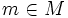$m \in M$ there exists an open neighbourhood$U$ of$M$ such that the map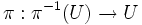$\pi:\pi^{-1}(U) \to U$ is the same as the projection map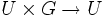$U \times G \to U$.

Intuitively a principal$G$-bundle means a copy of$G$ at each point, varying smoothly with the point. However, the copy of$G$ at each point, does not have any natural "origin" or identity element.

## Facts

### Reduction of structure group

Further information: Reduction of structure group

If$H$ is a subgroup of$G$ and$M$ hs a principal$G$-bundle$P$, we may be able to find a subbundle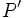$P'$ that is a principal$H$-bundle. Intuitively, what we are doing is that at each$m \in M$, we are choosing a subset of the copy of$G$ that is the orbit of a single point under the$H$-action. The tricky part is to make a choice that varies smoothly with the point.

The process of finding a subbundle that is principal for a subgroup is termed reduction of the structure group.

### Lifting of structure group

Further information: Lifting of structure group

Let$G$ be a Lie group,$N$ a normal subgroup that is also a Lie subgroup, and$G/N$ the quotient group. Then any principal$G$-bundle gives rise to a$G/N$-bundle as follows: if$\pi:P \to M$ is the$G$-bundle, then the$G/N$-bundle is simply$P$ modulo the action of$N$. The fibre at each point is the quotient space by$N$.

The question of lifting the structure group is the other way around: given a principal bundle over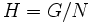$H = G/N$, find a principal$G$-bundle that would give rise to this on quotienting by$N$.

### Vector bundles as principal bundles

A vector bundle on a differential manifold can be viewed as a principal bundle with structure group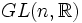$GL(n,\R)$. If$M$ is the differential manifold, the fibre at$m \in M$ is the set of all ordered bases for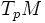$T_pM$.

### Connections on principal bundles

Further information: Connection on principal bundle

Typically the term connection is used for a vector bundle on a differential manifold, and it is intended to be a rule that allows us to differentiate sections of the vector bundle alogn vector fields (viz, sections of the tangent bundle).

We can generalize this to define a notion of connection on a principal bundle. Fill this in later